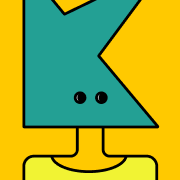# CML- Chapter 7: Decimals

\$31880918404805
0 ratings
Chapter seven covers a variety of topics including place value, decimals, decimal computation, ratio and proportion, percents, and real numbers. These topics are related in that they are topics children study in the upper elementary grades 4, 5 and 6, and several of them are mathematically related, especially place value less than one, decimals, and percents. These topics are also related in that they require children to do higher level thinking such as proportional reasoning. The topics in this chapter are interconnected and studying one concept may involve using or knowing another concept in this chapter. For example, using decimal numbers and performing decimal computations are frequently required in solving proportions
495 KB
35 pages
\$3

0 ratings